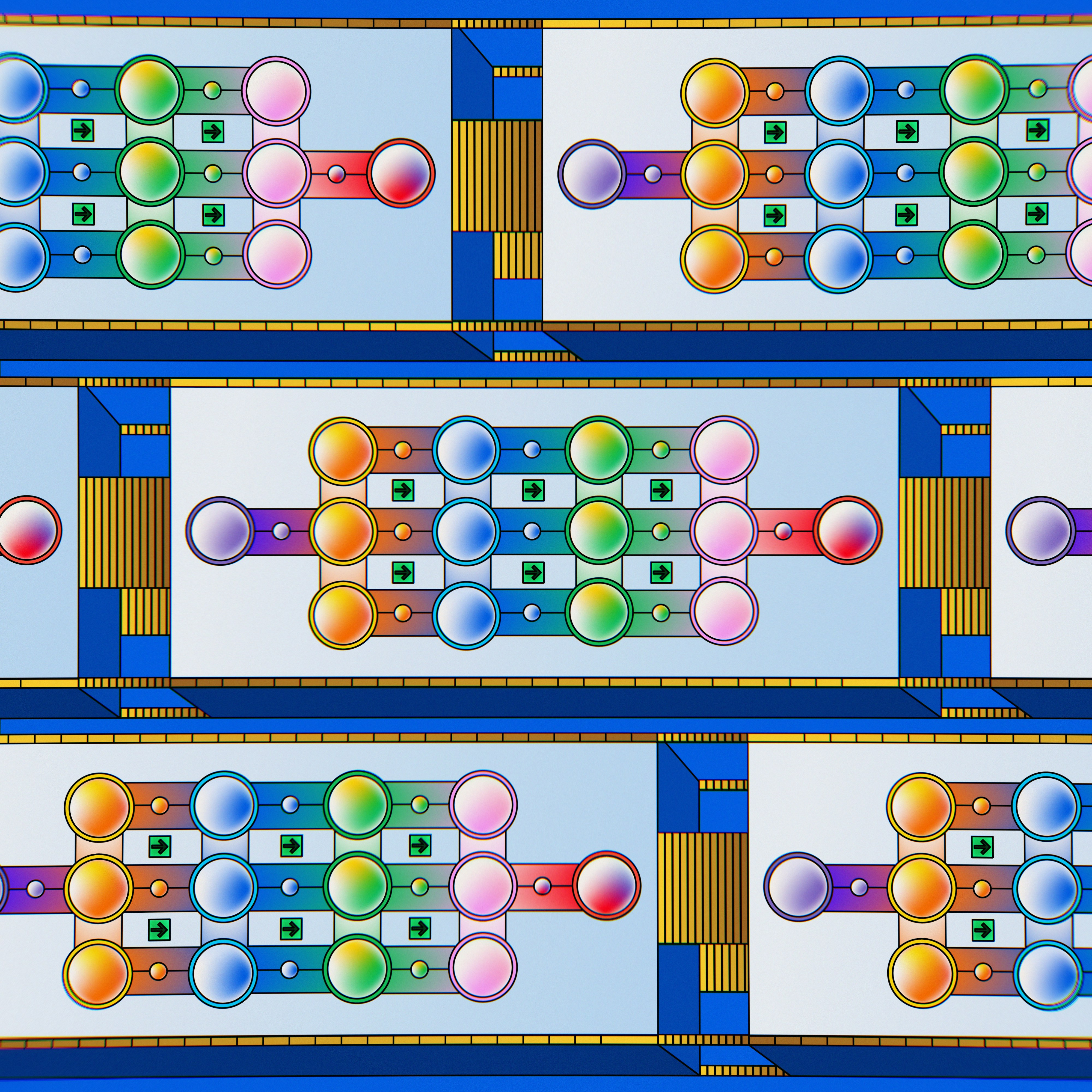## 机器学习：回归模型评价与检验

4108 字 · 342 阅读 · 2023 年 05 月 05 日### 知识点

• 拟合优度检验
• 变量显著性检验

$$\operatorname{MAE}(y, \hat{y})=\frac{1}{n} \sum_{i=1}^{n}\left|y_{i}-\hat{y}_{i}\right| \tag{1}$$

$$\textrm{MSE}(y, \hat{y} ) = \frac{1}{n} \sum_{i=1}^{n} (y_i - \hat{y})^{2} \tag{2}$$

$$\textrm{MAPE}(y, \hat{y} ) = \frac{\sum_{i=1}^{n}{|\frac{y_{i}-\hat y_{i}}{y_{i}}|}}{n} \times 100 \tag{3}$$

sklearn.metrics.mean_absolute_error(y_true, y_pred)  # MAE 计算
sklearn.metrics.mean_squared_error(y_true, y_pred)  # MSE 计算


### 拟合优度检验

$$\hat{y}_{i}=w x_{i}+b \tag{4}$$

「离差」进而可以分解为：

$$Y_{i}=y_{i}-\overline{y}=\left(y_{i}-\hat{y}_{i}\right)+\left(\hat{y}_{i}-\overline{y}\right)=e_{i}+\hat{Y}_{i} \tag{5}$$$$TSS=\sum_{i=1}^{n} Y_{i}^{2}=\sum_{i=1}^{n}\left(y_{i}-\overline{y}\right)^{2} \tag{6}$$
$$E S S=\sum_{i=1}^{n} \hat{Y}_{i}^{2}=\sum_{i=1}^{n}\left(\hat{y}_{i}-\overline{y}\right)^{2} \tag{7}$$
$$RSS=\sum_{i=1}^{n} e_{i}^{2}=\sum_{i=1}^{n}\left(y_{i}-\hat{y}_{i}\right)^{2} \tag{8}$$

$$TSS=\sum_{i=1}^{n}\left(y_{i}-\bar{y}\right)^{2} = \sum_{i=1}^{n}\left(\left(y_{i} - \hat{y}_{i}\right) + \left(\hat{y}_{i} -\bar{y}\right)\right)^{2}$$
$$=\sum_{i=1}^{n}\left(y_{i}-\hat{y}_{i}\right)^{2}+\sum_{i=1}^{n}\left(\hat{y}_{i}-\bar{y}\right)^{2}+2 \sum_{i=1}^{n}\left(y_{i}-\hat{y}_{i}\right)\left(\hat{y}_{i}-\bar{y}\right) \tag{9}$$

$$Q\left(b, w\right)=\min _{b, w} Q\left(b, w\right)=\sum_{i=1}^{n}\left(y_{i}-b-w x_{i}\right)^{2} \tag{10}$$

$$\frac{\partial Q}{\partial b}=-2 \sum_{i=1}^{n}\left(y_{i}-b-w x_{i}\right)=0 \tag{11}$$
$$\frac{\partial Q}{\partial w}=-2 \sum_{i=1}^{n} x_{i} \left(y_{i}-b-w x_{i}\right)=0 \tag{12}$$

$$\sum_{i=1}^{n}\left(y_{i}-\hat{y}_{i}\right)\left(\hat{y}_{i}-\bar{y}\right)=\sum_{i=1}^{n}\left(y_{i}-b-w x_{i}\right)\left(b+w x_{i}-\bar{y}\right)$$
$$=b\sum_{i=1}^{n}\left(y_{i}-b-w x_{i}\right) + w\sum_{i=1}^{n}x_{i}\left(y_{i}-b-w x_{i}\right)-\bar{y}\sum_{i=1}^{n}\left(y_{i}-b-w x_{i}\right) \tag{13}$$

$$\sum_{i=1}^{n}\left(y_{i}-\bar{y}\right)^{2}=\sum_{i=1}^{n}\left(y_{i}-\hat{y}_{i}\right)^{2}+\sum_{i=1}^{n}\left(\hat{y}_{i}-\bar{y}\right)^{2} \tag{14}$$

$$TSS=ESS+RSS \tag{15}$$

$$R^{2}=\frac{ESS}{TSS}=1-\frac{RSS}{TSS} \tag{16}$$

import numpy as np

# 生成 2 组 x 相同的示例数据
x = np.array([4, 8, 12, 25, 32, 43, 58, 63, 69, 79]).reshape(-1, 1)
y1 = np.array([9, 17, 23, 51, 62, 83, 115, 125, 137, 159]).reshape(-1, 1)
y2 = np.array([20, 33, 50, 56, 42, 31, 33, 46, 65, 75]).reshape(-1, 1)

from matplotlib import pyplot as plt
%matplotlib inline

# 两组数据分别绘制子图
fig, axes = plt.subplots(1, 2, figsize=(12, 4))
axes.scatter(x, y1)
axes.scatter(x, y2)

from sklearn.linear_model import LinearRegression

model1 = LinearRegression()  # 线性模型 1
model1.fit(x, y1)

model2 = LinearRegression()  # 线性模型 2
model2.fit(x, y2)

# 绘制拟合直线到原散点图中
fig, axes = plt.subplots(1, 2, figsize=(12, 4))
# 模型 1
axes.scatter(x, y1)
axes.plot([0, 90], [model1.intercept_,
model1.coef_ * 90 + model1.intercept_], 'r')
# 模型 2
axes.scatter(x, y2)
axes.plot([0, 90], [model2.intercept_,
model2.coef_ * 90 + model2.intercept_], 'r')


from sklearn.metrics import r2_score

# 分别传入真实观测值和模型预测值
r2_score(y1, model1.predict(x)), r2_score(y2, model2.predict(x))


# 使用公式计算方法验证 model1 计算结果
rss = np.sum(pow((y1 - model1.predict(x)), 2))
tss = np.sum(pow((y1 - np.mean(y1)), 2))


plt.scatter(x, y1)
plt.plot([0, 90], [200, -2 * 90 + 200], 'r')  # 线性函数为：y = -2x + 200
plt.title("r2_score: {}".format(r2_score(y1, -2 * x + 200)))


### 变量显著性检验

import statsmodels.api as sm

x = sm.add_constant(x)  # 添加截距项系数 1
model = sm.OLS(endog=y2, exog=x)  # 普通最小二乘法, endog 因变量, exog 自变量
results = model.fit()
results.params  # 输出拟合后系数

x


import warnings
warnings.filterwarnings('ignore')

results.summary2(alpha=0.05)  # 输出模型摘要, 95% 置信度


- Coef. Std.Err. t P>abs(t) [0.025] [0.975]
const 30.1297 8.1820 3.6824 0.0062 11.2620 48.9975
x1 0.3809 0.1742 2.1865 0.0602 -0.0208 0.7827

### 系列文章•